Rules for Developing a Model

 Since mathematical models (regression models) are often used to predict the relationship between paired data elements, it is important to understand how to choose a model that will be a "good fit" for the particular data set.   There are several things to keep in mind when attempting to develop a model that will be a "good fit":1.  Visually compare the graph of the data to the graph of the model.  (Look for a pattern from the graph.)Prepare a scatter plot and examine the graph.  Look to see which regression model appears to best represent the scatter plot graph.  Know the general shapes of the regression models.  When trying to select a model, choose only those models that appear to fit the observed points reasonably well.  Extend the WINDOW to see how the regression equation behaves at higher x-values.

Linear based regression models:
(Other representations of these shapes may also exist due to the different natures of the data.)

 Linear  y = ax + b (or y = a + bx) Logarithmic  y = a + b lnx Exponential y = abx Power  y = axbDoes the plotted data resemble a straight line? • The slope may be either positive or negative. • Linear associations are the most popular because they are easy to read and interpret. NOTE: See LinReg(ax+b) versus LinReg(a+bx) for an explanation of the "differences" between these two choices on the graphing calculator.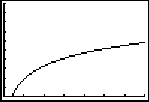Does the plotted data ascend rapidly at the left but level off toward the right? Remember the shape of the natural logarithmic function crossing the x-axis at one and domain x > 0.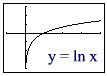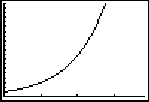Does the plotted data appear to grow (or decline) by percentage increases (decreases)? • Useful for values that grow by percentage increases. • Often deals with growth of populations, bacteria, radio-active decay, etc. Remember the shape of the exponential function, crossing the y-axis at one and range y > 0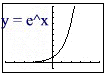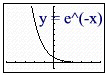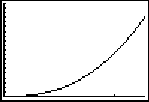Does the plotted data possess characteristics not seen in the first three models?  Not a straight line, but a more gradual change than exponential? •  Power functions are of the form y = axb. Remember the nature of such graphs when the exponent is odd and even.   First quadrant: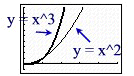Outside first quadrant: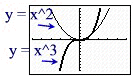Other regressions:

 Quadratic y = ax2 + bx + c Logistic y = c/(1 + ae -bx) Cubic Quartic Sinusoidal y = asin(bx + c) + d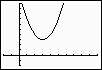Modified version of power model.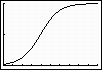S-shaped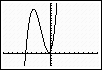Modified version of power model.Modified version of power model.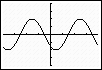Remember the periodic nature of such graphs.

2.  Calculate a correlation coefficient, r (for some models).
The correlation coefficient measures the strength and the direction of a linear relationship between two variables.  A value of | r | near one may indicate a "good fit".

3.  Calculate a coefficient of determination, r2 (R2).
The coefficient of determination represents the percent of the data that is the closest to the line of best fit.  For example, if r = 0.922, then r 2 = 0.850, which means that 85% of the total variation in y can be explained by the linear relationship between x and y (as described by the regression equation).  The other 15% of the total variation in y remains unexplained.

Do not place too much importance on small differences between r2 values, such as r2 = 0.987 and r2 = 0.984.  Also, keep in mind that r, r2 and R2 values cannot be directly compared when calculating certain regression models.

4.  Examine the residuals.
Examine the scatter plot of the residuals, which depicts the measure of the signed distances between the actual data values and the outputs predicted by the model.  A good linear model has residuals that are near zero and are randomly distributed.

Is your choice realistic?  Don't use a model that will lead to predicted values that are totally unrealistic.

"The best choice (of a model) depends on the set of data being analyzed and requires an exercise in judgment, not just computation."
"Modeling the US Population" by Shelly Gordon

 IMPORTANT!! Each time you compute a new regression model, the previously computed model is lost. If you compute a linear model and then compute an exponential model, your information pertaining to the linear model is lost.  You will need to re-compute the linear information if you wish to investigate that model further.Finding Your Way Around TABLE of  CONTENTS